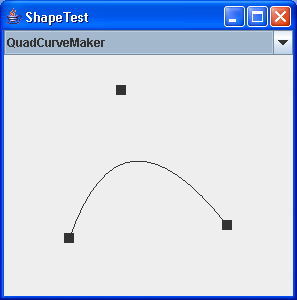# Hypnosis Spiral : Shape « 2D Graphics GUI « Java

Hypnosis Spiral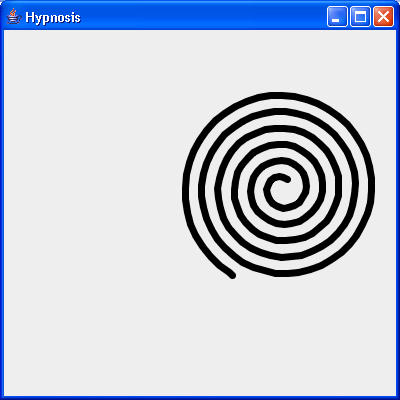```
/*
* This code is from the book Java Examples in a Nutshell, 2nd Edition.
* It is provided AS-IS, WITHOUT ANY WARRANTY either expressed or implied.
* You may study, use, and modify it for any non-commercial purpose.
* You may distribute it non-commercially as long as you retain this notice.
* For a commercial use license, or to purchase the book (recommended),
* visit http://www.davidflanagan.com/javaexamples2.
*/

import java.awt.BasicStroke;
import java.awt.BorderLayout;
import java.awt.Color;
import java.awt.Graphics;
import java.awt.Graphics2D;
import java.awt.Rectangle;
import java.awt.RenderingHints;
import java.awt.event.ActionEvent;
import java.awt.event.ActionListener;
import java.awt.image.BufferedImage;

import javax.swing.JComponent;
import javax.swing.JFrame;
import javax.swing.Timer;

/**
* A Swing component that smoothly animates a spiral in a hypnotic way.
*/
public class Hypnosis extends JComponent implements ActionListener {
double x, y; // The center of the spiral

double r1, r2; // The inner and outer radii of the spiral

double a1, a2; // The start and end angles of the spiral

double deltaA; // How much the angle changes each frame

double deltaX, deltaY; // The trajectory of the center

float linewidth; // How wide the lines are

Timer timer; // The object that triggers the animation

BufferedImage buffer; // The image we use for double-buffering

Graphics2D osg; // Graphics2D object for drawing into the buffer

public Hypnosis(double x, double y, double r1, double r2, double a1,
double a2, float linewidth, int delay, double deltaA,
double deltaX, double deltaY) {
this.x = x;
this.y = y;
this.r1 = r1;
this.r2 = r2;
this.a1 = a1;
this.a2 = a2;
this.linewidth = linewidth;
this.deltaA = deltaA;
this.deltaX = deltaX;
this.deltaY = deltaY;

// Set up a timer to call actionPerformed() every delay milliseconds
timer = new Timer(delay, this);

// Create a buffer for double-buffering
buffer = new BufferedImage((int) (2 * r2 + linewidth),
(int) (2 * r2 + linewidth), BufferedImage.TYPE_INT_RGB);

// Create a Graphics object for the buffer, and set the linewidth
// and request antialiasing when drawing with it
osg = buffer.createGraphics();
osg.setStroke(new BasicStroke(linewidth, BasicStroke.CAP_ROUND,
BasicStroke.JOIN_ROUND));
osg.setRenderingHint(RenderingHints.KEY_ANTIALIASING,
RenderingHints.VALUE_ANTIALIAS_ON);
}

// Start and stop the animation by starting and stopping the timer
public void start() {
timer.start();
}

public void stop() {
timer.stop();
}

/**
* Swing calls this method to ask the component to redraw itself. This
* method uses double-buffering to make the animation smoother. Swing does
* double-buffering automatically, so this may not actually make much
* difference, but it is important to understand the technique.
*/
public void paintComponent(Graphics g) {
// Clear the background of the off-screen image
osg.setColor(getBackground());
osg.fillRect(0, 0, buffer.getWidth(), buffer.getHeight());

// Now draw a black spiral into the off-screen image
osg.setColor(Color.black);
osg.draw(new Spiral(r2 + linewidth / 2, r2 + linewidth / 2, r1, a1, r2,
a2));

// Now copy that off-screen image onto the screen
g.drawImage(buffer, (int) (x - r2), (int) (y - r2), this);
}

/**
* This method implements the ActionListener interface. Our Timer object
* calls this method periodically. It updates the position and angles of the
* spiral and requests a redraw. Instead of redrawing the entire component,
* however, this method requests a redraw only for the area that has
* changed.
*/
public void actionPerformed(ActionEvent e) {
// Ask to have the old bounding box of the spiral redrawn.
// Nothing else has anything drawn in it, so it doesn't need a redraw
repaint((int) (x - r2 - linewidth), (int) (y - r2 - linewidth),
(int) (2 * (r2 + linewidth)), (int) (2 * (r2 + linewidth)));

// Now animate: update the position and angles of the spiral

// Bounce if we've hit an edge
Rectangle bounds = getBounds();
if ((x - r2 + deltaX < 0) || (x + r2 + deltaX > bounds.width))
deltaX = -deltaX;
if ((y - r2 + deltaY < 0) || (y + r2 + deltaY > bounds.height))
deltaY = -deltaY;

// Move the center of the spiral
x += deltaX;
y += deltaY;

// Increment the start and end angles;
a1 += deltaA;
a2 += deltaA;
if (a1 > 2 * Math.PI) { // Don't let them get too big
a1 -= 2 * Math.PI;
a2 -= 2 * Math.PI;
}

// Now ask to have the new bounding box of the spiral redrawn. This
// rectangle will be intersected with the redraw rectangle requested
// above, and only the combined region will be redrawn
repaint((int) (x - r2 - linewidth), (int) (y - r2 - linewidth),
(int) (2 * (r2 + linewidth)), (int) (2 * (r2 + linewidth)));
}

/** Tell Swing not to double-buffer for us, since we do our own */
public boolean isDoubleBuffered() {
return false;
}

/** This is a main() method for testing the component */
public static void main(String[] args) {
JFrame f = new JFrame("Hypnosis");
Hypnosis h = new Hypnosis(200, 200, 10, 100, 0, 11 * Math.PI, 7, 100,
2 * Math.PI / 30, 3, 5);
f.setSize(400, 400);
f.show();
h.start();
}
}

```

### Related examples in the same category

 1 Creating Basic Shapes 2 fillRect (int, int, int, int) method draws a solid rectangle 3 Creating a Shape Using Lines and Curves 4 Combining Shapes 5 Draw rectangles, use the drawRect() method. To fill rectangles, use the fillRect() method 6 Draw line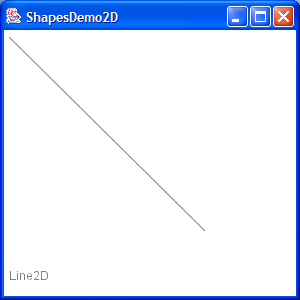7 Draw a Polygon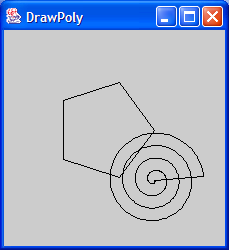8 Draw an oval outline 9 Draw a (Round)rectangle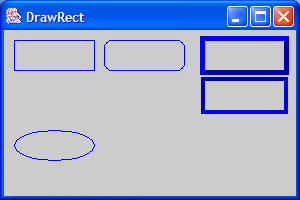10 Fill a polygon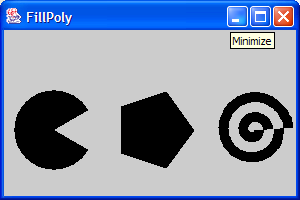11 Fill a solid oval 12 Fill a (Round)rectangle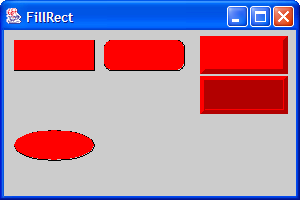13 Change font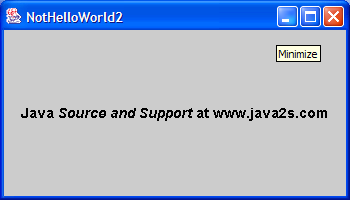14 Draw rectangle 2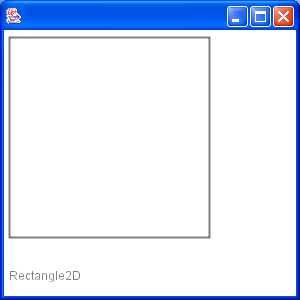15 Draw Arc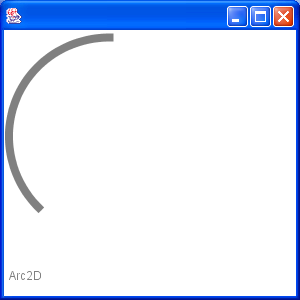16 Draw Ellipse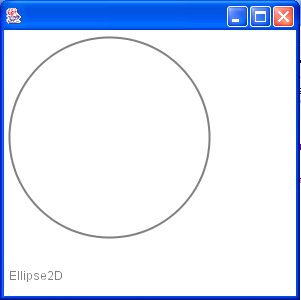17 Fill a Rectangle 2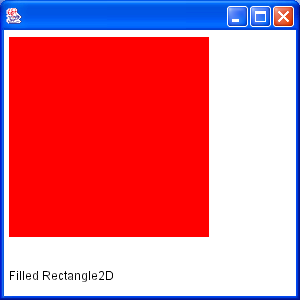18 Fill Arc 2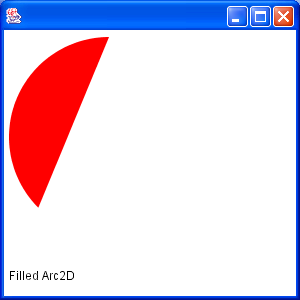19 Draw text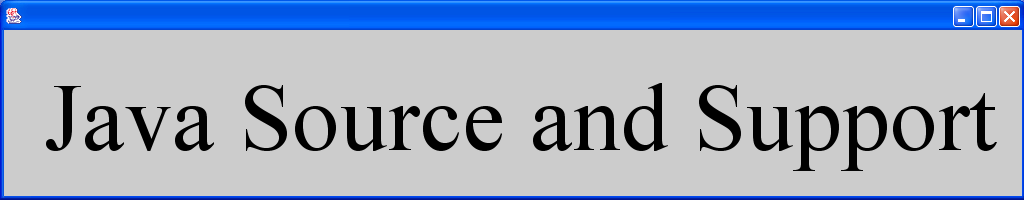20 Draw unicode string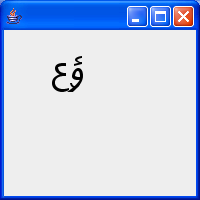21 Shape combine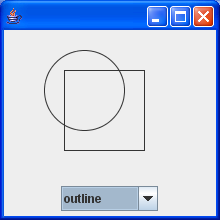22 Effects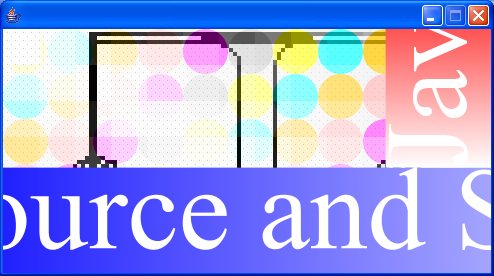23 Mouse drag and drop to draw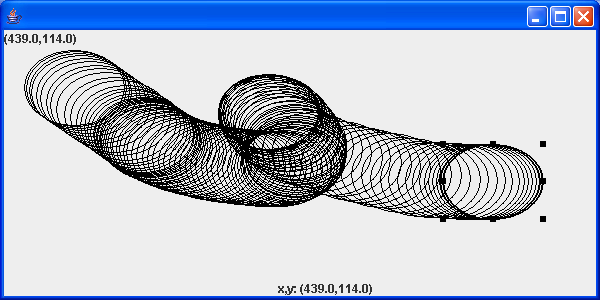24 Arc demonstration: scale, move, rotate, sheer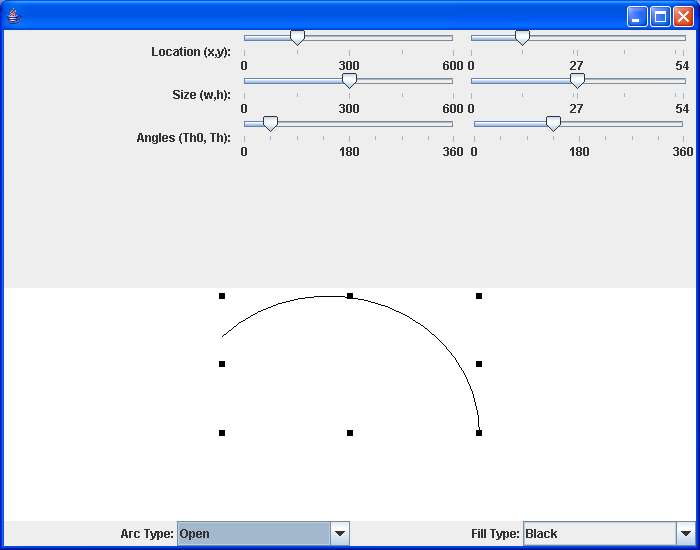25 GlyphVector.getNumGlyphs() 26 Resize a shape 27 Rectangle with rounded corners drawn using Java 2D Graphics API 28 Compares two ellipses and returns true if they are equal or both null. 29 Compares two lines are returns true if they are equal or both null. 30 Creates a diagonal cross shape. 31 Creates a diamond shape. 32 Creates a new Stroke-Object for the given type and with. 33 Creates a region surrounding a line segment by 'widening' the line segment. 34 Creates a triangle shape that points downwards. 35 Creates a triangle shape that points upwards. 36 Generate Polygon 37 Polygon with float coordinates. 38 Polyline 2D 39 Serialises a Shape object. 40 Tests two polygons for equality. If both are null this method returns true. 41 Union two rectangles 42 Calculate Intersection Clip 43 Draws a shape with the specified rotation about (x, y). 44 Checks, whether the given rectangle1 fully contains rectangle 2 (even if rectangle 2 has a height or width of zero!). 45 Reads a Point2D object that has been serialised by the writePoint2D(Point2D, ObjectOutputStream)} method. 46 Returns a point based on (x, y) but constrained to be within the bounds of a given rectangle. 47 RectListManager is a class to manage a list of rectangular regions. 48 Fill Rectangle2D.Double and Ellipse2D.Double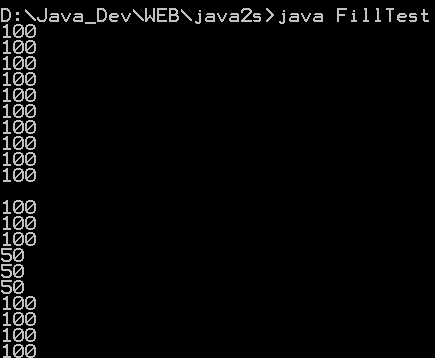49 This program demonstrates the various 2D shapes